• TECH INFO
• 用于Raspberry Pi的Sense HAT扩展板——操纵杆

TECH INFORaspberry Pi初学者指南

# 用于Raspberry Pi的Sense HAT扩展板——操纵杆

Sense HAT专为Astro Pi任务而设计，内置操纵杆是其中一个输入设备。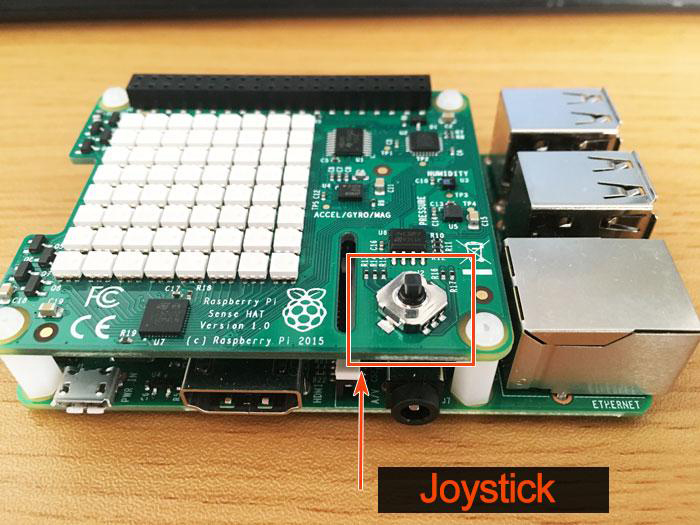## 操纵杆的输入值

Sense HAT操纵杆映射到四个键盘光标键，操纵杆中键单击映射为Return（返回）键。这意味着移动操纵杆与按下对应键的效果完全相同。请记住，下向是指HDMI端口朝下的方向。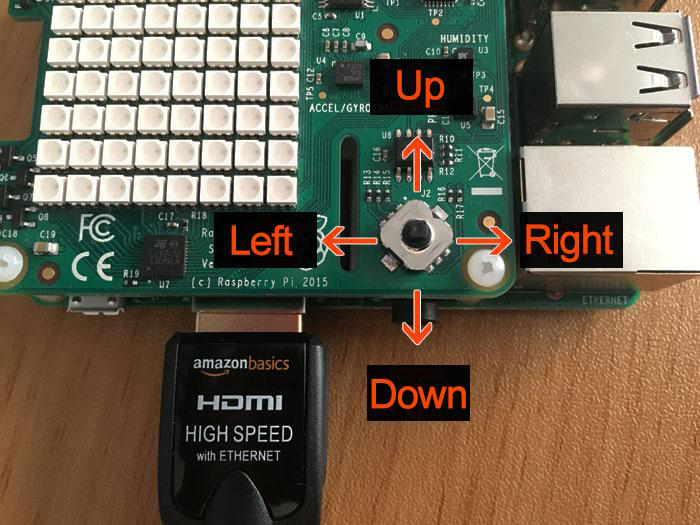``````import pygame

from pygame.locals import *
from sense_hat import SenseHat

pygame.init()
pygame.display.set_mode((640, 480))
sense = SenseHat()
sense.clear()

running = True

while running:
for event in pygame.event.get():
print(event)
if event.type == QUIT:
running = False
print("BYE")
``````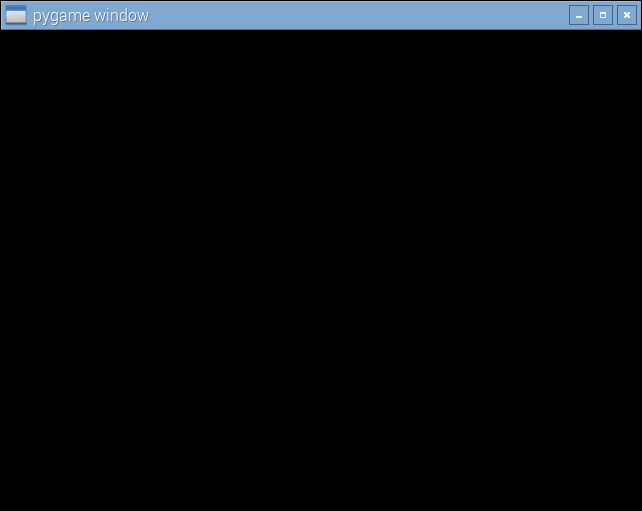Pygame – 维基百科

Pygame是一个由多个Python模块组成的跨平台套件，专为视频游戏编写而设计。它包括计算机图形库和声音库，旨在与Python编程语言一起使用。

pygame.event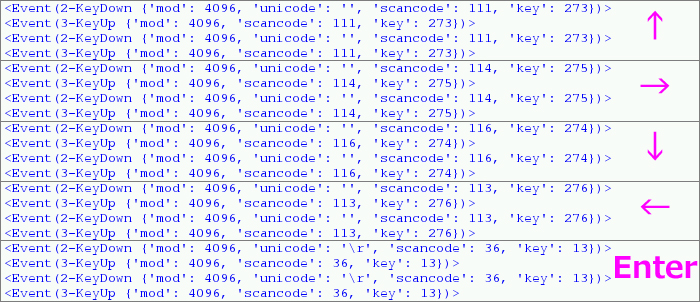## 操纵杆和LED显示器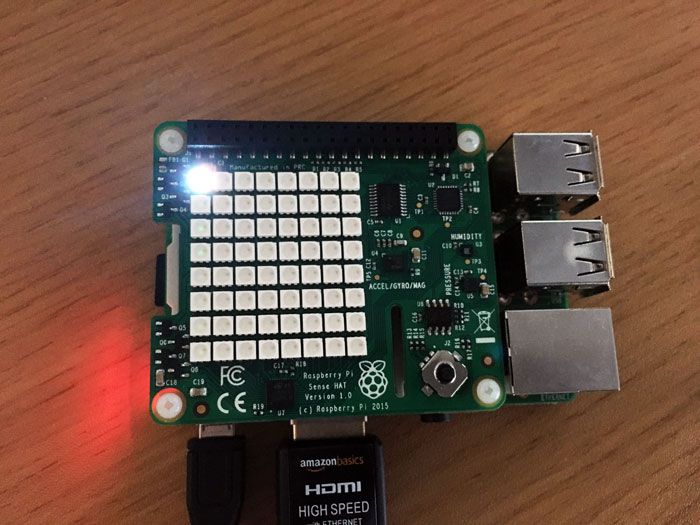• 关闭点亮的LED
• 点亮目标LED

Set_pixel

``````#Lights up (white)
sense.set_pixel(0, 0, 255, 255, 255)
#Lights off (black)
sense.set_pixel(0, 0, 0, 0, 0)
``````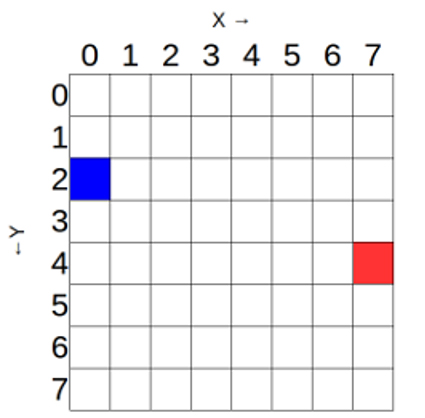LED显示屏的坐标起始于左上角——此处的坐标为0，如图5所示。操纵杆的动作以及X坐标和Y坐标的变化如下图所示。

 操纵杆动作 坐标变化 向上 y-1 向下 y+1 向左 x-1 向右 x+1

ValueError: Y position must be between 0 and 7

``````import pygame

from pygame.locals import *
from sense_hat import SenseHat

pygame.init()
pygame.display.set_mode((640, 480))

sense = SenseHat()
sense.clear()

running = True

x = 0
y = 0
sense.set_pixel(x, y, 255, 255, 255)

while running:
for event in pygame.event.get():
if event.type == KEYDOWN:
sense.set_pixel(x, y, 0, 0, 0)  # Black 0,0,0 means OFF

if event.key == K_DOWN and y < 7:
y = y + 1
elif event.key == K_UP and y > 0:
y = y - 1
elif event.key == K_RIGHT and x < 7:
x = x + 1
elif event.key == K_LEFT and x > 0:
x = x - 1

sense.set_pixel(x, y, 255, 255, 255)
if event.type == QUIT:
running = False
print("BYE")
``````

## 我们来制作一个游戏！

Sense HAT Pong | Raspberry Pi 学习资源

Pong是一款老式的复古游戏。在该游戏中，玩家用一个球拍/条块杆击打移动的球。

/home/pi/nas/pong.py

``````from time import sleep
from sense_hat import SenseHat
import curses

sense = SenseHat()
sense.clear(0,0,0)

screen = curses.initscr()
curses.cbreak()
curses.noecho()

y = 4
ball_position=[6,3]
ball_speed=[-1,-1]

def drawbat():
sense.set_pixel(0,y,255,255,255)
sense.set_pixel(0,y+1,255,255,255)
sense.set_pixel(0,y-1,255,255,255)

def moveball():
global game_over
while True:
sleep(0.15)

sense.set_pixel(ball_position,ball_position,0,0,0)

ball_position += ball_speed
ball_position += ball_speed

if ball_position == 0 or ball_position == 7:
ball_speed = -ball_speed
if ball_position == 7:
ball_speed = -ball_speed
if ball_position == 1 and y-1 <= ball_position <= y+1:
ball_speed = -ball_speed
if ball_position == 0:
break

sense.set_pixel(ball_position,ball_position,0,0,255)

game_over = True

game_over = False

while not game_over:
drawbat()

key = screen.getch()
sense.clear()
if key == curses.KEY_UP:
if y > 1:
y -= 1

if key == curses.KEY_DOWN:
if y < 6:
y += 1

sense.show_message("You Lose", text_colour=(255,0,0))

curses.nocbreak()
curses.echo()
curses.endwin()
``````

python3 /home/pi/nas/pong.py

/home/pi/nas/pong_joy.py

``````from time import sleep
from sense_hat import SenseHat
import pygame
from pygame.locals import *

sense = SenseHat()
sense.clear(0,0,0)

pygame.init()
pygame.display.set_mode((320, 240))
y = 4
ball_position=[6,3]
ball_speed=[-1,-1]

def drawbat():
sense.set_pixel(0,y,255,255,255)
sense.set_pixel(0,y+1,255,255,255)
sense.set_pixel(0,y-1,255,255,255)

def moveball():
global game_over
while True:
sleep(0.5)

sense.set_pixel(ball_position,ball_position,0,0,0)

ball_position += ball_speed
ball_position += ball_speed

if ball_position == 0 or ball_position == 7:
ball_speed = -ball_speed
if ball_position == 7:
ball_speed = -ball_speed
if ball_position == 1 and y-1 <= ball_position <= y+1:
ball_speed = -ball_speed
if ball_position == 0:
break

sense.set_pixel(ball_position,ball_position,0,0,255)

game_over = True

game_over = False

while not game_over:
drawbat()

for event in pygame.event.get():
if event.type == KEYDOWN:
if event.key == K_UP and y > 1:
sense.set_pixel(0,y+1,0,0,0)
y -= 1
if event.key == K_DOWN and y < 6:
sense.set_pixel(0,y-1,0,0,0)
y += 1

sense.show_message("You Lose", text_colour=(255,0,0))
``````

python3 /home/pi/nas/pong_joy.py

## 总结